# Gear ratios and compound gear ratios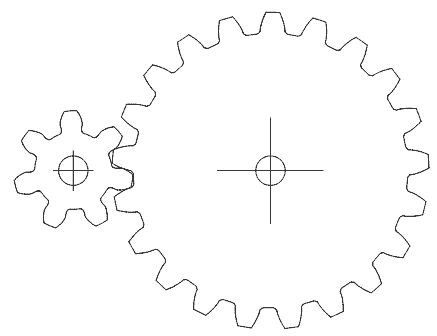## Working out simple gear ratios (two gears)

A feature often requested in my gear program is that it should calculate and display the gear ratio.

The reason it does not have this feature is that the gear ratio is also the tooth count ratio (of the two gears), and that is a value that the user has to enter.

At left, the two meshing gears with 7 teeth and 21 teeth will have a ratio of 7:21 (which is the same as 1:3). That is to say, the 7-tooth gear will turn 3 times for ever one turn of the 21-tooth gear. The logic is simple, each gear needs to rotate by the same number of teeth for them to mesh, so the 7-tooth gear, having one third the teeth, needs to turn three times as much

Determining what gears you need
Suppose you have a motor that turns 1200 RPM (revolutions per minute), and you need to turn something at 500 RPM.
The ratio you need is 500:1200, or 5:12. However, simple gears with only 5 teeth tend to run a bit rough, so your best bet is to make (or obtain) gears with 10 and 24 teeth.

## Determining compound gear ratios (multiple stages)

When a gear train has multiple stages, the gear ratio for the overall gearing system is the product of the individual stages.

For example, for the gear at left the blue gears are 7 and 21 teeth, while the green gears are 9 and 30 teeth. Thus, the first gear ratio is 7:21 and the second is 9:30. Multiplying the two together gives (7x9):(21x30) = 63 : 630, which is 1:10. So the big green gear will make 1 turn for every 10 turns of the small blue gear.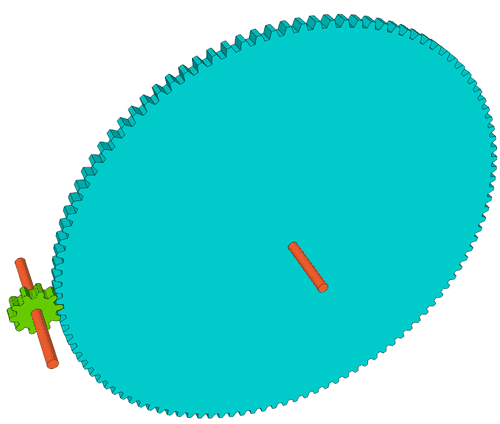Working out what gears you need for designing multiple stage gearing
Any gear ratio that can be achieved by multiple stages of gearing can also be produced by single stage gearing, but for large gear ratios, the large gear can become unwieldy.

There are many ways to achieve a given reduction with multiple stages, but how to decide what tooth counts to use for the gears?

Suppose we need a gear ratio that is 1:11, and we want the smallest gear to have no fewer than 10 teeth. We could do this with a 10-tooth and a 110-tooth gear. Lets write the ratio as

10:110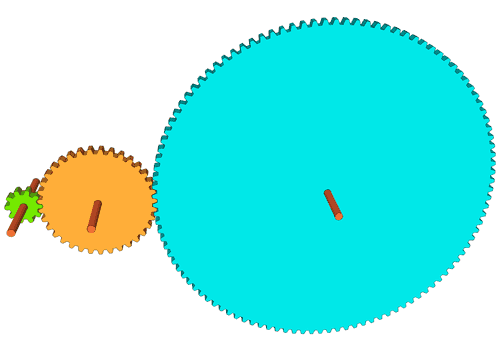Now, let's imagine we put another gear in between those. Let's imagine putting a 35 tooth gear between the 10 and the 110 tooth gears. The gear ratio between the 10 and the 110 tooth gears will still be the same, though the 10 and 110 tooth gears will now rotate in the same direction, whereas before they turned in opposite directions.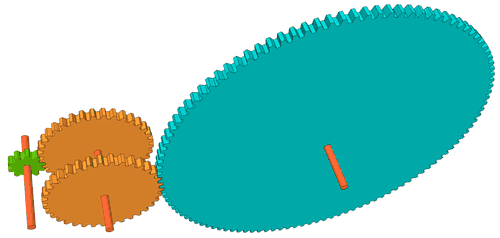With the 35-tooth gear in between, we can now think of now having a 10:35 tooth reduction, followed by a 35:110 tooth reduction.We can reduce the 35:110 to 7:22, but if we don't want any gears smaller than 10 teeth, we need to double that to 14:44. So we can now make our 1:11 gearing with the following stages:

10:35   and  14:44

Total number of teeth between the two stages is 103 teeth, vs 120 for the original version. but more importantly, this gear set is smaller.

Common denominators are very important, and it may be necessary to pick a different intermediate tooth count to make reduction possible.. If, however, we wanted an 11 : 127 gear ratio, the only way to get that exact ratio would be with an 11 tooth and a 127 tooth gear (or multiples thereof), because both are prime numbers that can't be factored.

On further consideration of the above 1:11 gearing example, we could have done even better if we instead started with 1:11 as 12:132. We could then write this as 12:44 and 44:132, and the 44:132 is a 1:3 ratio, which we could also make a 10:30. That would leave us with 12:44 and 10:30, which adds up to just 96 teeth total. We could also swap the two large gears if we wanted to. For example, 10:44 and 12:30 multiplied together also produce a 1:11 ratio.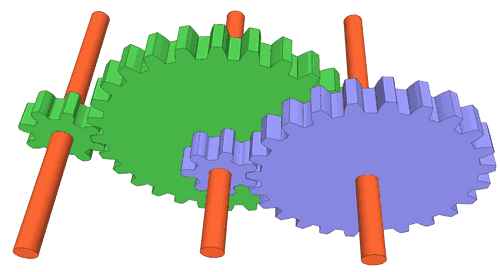Design example 2: Gears for clock face hour and minute hands

Suppose we want to make a 1:12 reduction for a clock. 12 factors into 4 and 3, so we could do a 1:4 and a 1:3 reduction. but let's see if we can make the two ratios closer to each other.

Let's multiply both sides by 8, so our 1:12 ratio becomes 8:96.
To get the reduction ratio for both gears, we want each gear ratio to be about the square root of 12, which is about 3.464. Now 8 * 3.464 is 27.7. So lets try 28 teeth for the intermediate gear.

So we can write 8:28:96 or 8:28 and 28:96 We can divide the right side by 4, so we get 8:28 and 7:24

It doesn't always work out so nicely. Sometimes one side or the other doesn't have any common divisors, so you may need to try different values for the intermediate.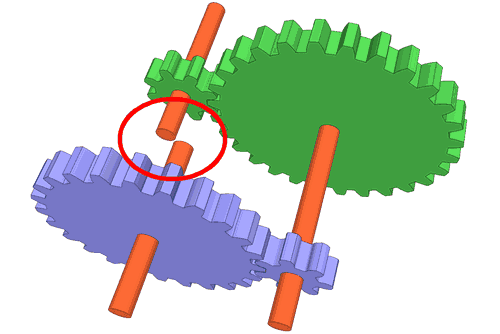For a clock, the hours and minute hands have to be concentric, so both of these gear pairs have to have the same shaft spacing. If we use the same tooth pitch for both gears, the shafts will not line up.Using my gear generator program, I can just enter the shaft spacing, and the program will recalculate the tooth size accordingly. I used 8 cm for both sets.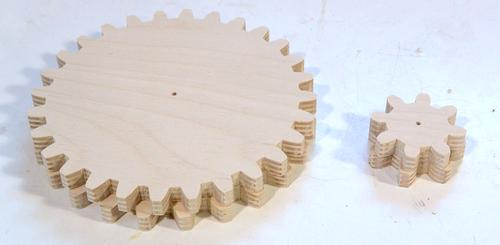I cut the gears out of some 10 mm thick plywood on the bandsaw.

If I place them on top of each other, the two sets of gears look almost identical, but they have slightly different ratios, and the teeth on one set are slightly larger.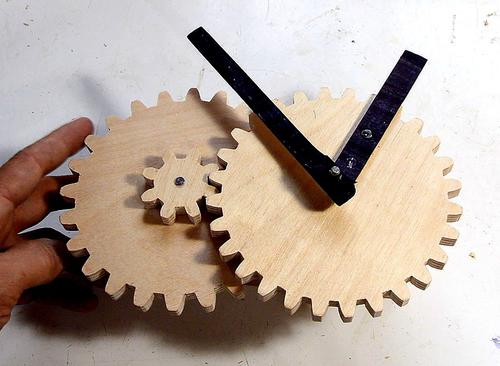A shaft through the larger gear on the right couples to the smaller 7-tooth gear behind it, and the shorter "hours" hand is screwed directly to the large gear.

Now, if I had a timer motor that turned 1 turn per hour, I could make a really big clock with these gears.

"Build a clock" is suggestion I often get. Maybe one of these days I will build one, maybe not. No need to suggest it at any rate, because the thought has certainly occurred to me :)

Back to my Woodworking website.